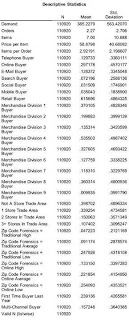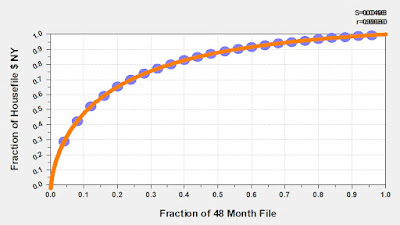## September 13, 2010

### Digital Profiles Part B: Diagnostics And MathWelcome to the next part in our series about Digital Profiles!

Recall in Part A that we created a dataset, a spreadsheet if you will. The spreadsheet has one row per customer, and has a series of columns that describe how the customer behaves.

Next, I create a table that shows the average value and standard deviation for each variable. This will be used later in the methodology to score each customer prior to segment assignment. The table is illustrated here on the left hand side of this blog post.

Now, things get a little complicated, and for good reason!

I am about to use a methodology called "Factor Analysis" or "Principal Components Analysis". Essentially, I want to ask the computer to combine any/all similarities in the database. Maybe all store customers buy from merchandise division #3, while all e-mail customers buy from merchandise division #8. If that is the case, then the methodology finds those similarities, and creates "factors" that are combinations of all of the similarities in the data.

Ok, here's where the statistician-elite jump all over me for violating 22,493 different assumptions. They'll tell me that I cannot us
e 1/0 variables in the analysis, they'll come up with a ton of reasons why my work is garbage.

Horsefeathers!

Listen, I'm not trying to protect a cancer patient from an experimental drug that may cause devastating side effects ... I'm simply trying to understand customer behavior better, and then convert that understanding into a series of pr
ofiles/segments that can be utilized in an actionable manner.

The factor analysis yields a rotated component matrix. I look for any value below - 0.200 or above 0.200. When I see this, I know that the variable with that coefficient plays a role in that factor.In most cases, the first four factors capture enough relevant information for a Digital Profile analysis.

In this case, the following attributes were important in the first factor.
• # of Orders
• Merchandise Division 5
• Merchandise Division 7
The second factor listed these attributes as being important.
• Number of Orders
• Price per Item (Negative, meaning low-cost items are favored)
• Items per Order
• Merchandise Division 4
• Merchandise Division 5
• Merchandise Division 6
• Merchandise Division 8 (negative)
• First Time Buyer (negative, meaning existing customers are favored)
The third factor listed these attributes as being important.
• Number of Orders
• Price per Item (high-cost items are favored)
• Merchandise Division 3
• Merchandise Division 5
• Merchandise Division 6
• Merchandise Division 8
• Merchandise Division 9
The fourth factor listed these attributes as being important.
• Number of Orders
• Price per Item (negative, meaning low-cost items are favored)
• Items per Order
• Merchandise Division 1
• Merchandise Division 2
• Merchandise Division 3
• Merchandise Division 4 (negative)
• Merchandise Division 5 (negative)
So, each factor describes something interesting about customers.

Now, here's where I go and once again ruin any remaining reputation I have with MS-level statisticians.

Each factor results in a score ... a standardized variable (mean = 0), with positive values reflecting the presence of the attributes listed above, a negative value representing a lack of the attributes listed above.

I run a quick analysis on each factor, identifying the median value of each factor. The median value should be reasonably close to zero, but seldom is with this kind of marketing data.
• Factor 1 Median = - 0.542.
• Factor 2 Median = 0.064.
• Factor 3 Median = - 0.193.
• Factor 4 Median = - 0.295.
Why did I do this?

Well, this is going to form the basis of the segmentation strategy.

I create a new variable, called "DP1". If factor 1 > - 0.542, set DP1 = 1, otherwise, set it equal to 0.

I create a new variable, called "DP2". If factor 2 > 0.064, set DP2 = 1, otherwise, set it equal to 0.

I create a new variable, called "DP3". If factor 3 > - 0.193, set DP3 = 1, otherwise, set it equal to 0.

I create a new variable, called "DP4". If factor 4 > - 0.295, set DP4 = 1, otherwise, set it equal to 0.

Ok, still with me? Good!

The final step is to evaluate every single combination of DP1, DP2, DP3, and DP4 values. Since each variable is a 1/0 indicator, there are 2*2*2*2 = 16 combinations. These, ladies and gentlemen, are the Digital Profiles!

If (DP1 = 1) and (DP2 = 1) and (DP3 = 1) and (DP4 = 1) Digital Profile = 1.
If (DP1 = 1) and (DP2 = 1) and (DP3 = 1) and (DP4 = 0) Digital Profile = 2.
If (DP1 = 1) and (DP2 = 1) and (DP3 = 0) and (DP4 = 1) Digital Profile = 3.
If (DP1 = 1) and (DP2 = 1) and (DP3 = 0) and (DP4 = 0) Digital Profile = 4.
If (DP1 = 1) and (DP2 = 0) and (DP3 = 1) and (DP4 = 1) Digital Profile = 5.
If (DP1 = 1) and (DP2 = 0) and (DP3 = 1) and (DP4 = 0) Digital Profile = 6.
If (DP1 = 1) and (DP2 = 0) and (DP3 = 0) and (DP4 = 1) Digital Profile = 7.
If (DP1 = 1) and (DP2 = 0) and (DP3 = 0) and (DP4 = 0) Digital Profile = 8.
If (DP1 = 0) and (DP2 = 1) and (DP3 = 1) and (DP4 = 1) Digital Profile = 9.
If (DP1 = 0) and (DP2 = 1) and (DP3 = 1) and (DP4 = 0) Digital Profile = 10.
If (DP1 = 0) and (DP2 = 1) and (DP3 = 0) and (DP4 = 1) Digital Profile = 11.
If (DP1 = 0) and (DP2 = 1) and (DP3 = 0) and (DP4 = 0) Digital Profile = 12.
If (DP1 = 0) and (DP2 = 0) and (DP3 = 1) and (DP4 = 1) Digital Profile = 13.
If (DP1 = 0) and (DP2 = 0) and (DP3 = 1) and (DP4 = 0) Digital Profile = 14.
If (DP1 = 0) and (DP2 = 0) and (DP3 = 0) and (DP4 = 1) Digital Profile = 15.
If (DP1 = 0) and (DP2 = 0) and (DP3 = 0) and (DP4 = 0) Digital Profile = 16.

We're there!!!!

Each customer has been assigned a Digital Profile, one of sixteen combinations of variables created from the Factor Analysis.

When I score files in the future, I use the standardized variables, created from the mean and standard deviation of each variable in the first table in this post, and use the Component Score Coefficient Matrix (I know, really, REALLY geeky) to calculate each factor.

Up Next: We'll begin to look at the composition of each Digital Profile!

1.Kevin:

Good stuff!

Suggestion: Include links to previous articles, Wikipia definitions of the main terms.

It often helps remind me to check the citations for the articles inside Wikipedia for new developments.

Michael

2.I'm probably not going down the Wikipedia route, but I will consider posting a series of links.

Thanks!
Kevin

3.Anonymous2:45 AM

it's very useful post thanks for sharing it

www.tasflowrance.com